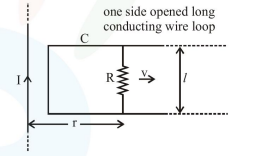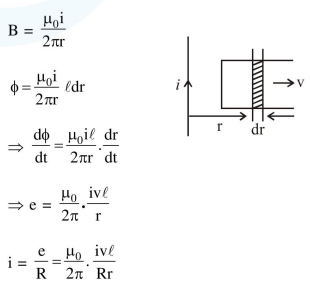# An infinitely long straight wire carrying current I,

Question:

An infinitely long straight wire carrying current I, one side opened rectangular loop and a conductor C with a sliding connector are located in the same

plane, as shown in the figure. The connector has length $l$ and resistance R. It slides to the right with a velocity v. The resistance of the conductor and

the self inductance of the loop are negligible. The induced current in the loop, as a function of separation $r$, between the connector and the straight

wire is :1. $\frac{\mu_{0}}{\pi} \frac{\mathrm{Iv} l}{\mathrm{Rr}}$

2. $\frac{\mu_{0}}{2 \pi} \frac{\mathrm{Iv} l}{\mathrm{Rr}}$

3. $\frac{2 \mu_{0}}{\pi} \frac{\mathrm{Iv} l}{\mathrm{Rr}}$

4. $\frac{\mu_{0}}{4 \pi} \frac{\mathrm{Iv} l}{\mathrm{Rr}}$

Correct Option: , 2

Solution: